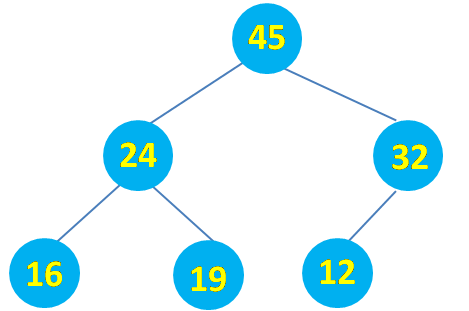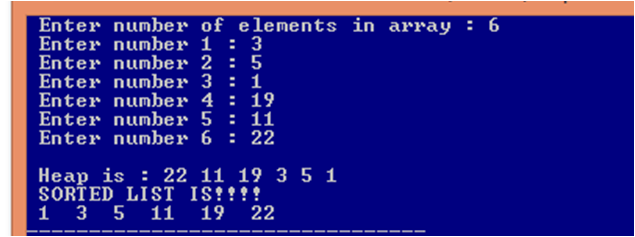# Heap Sort Program In C

## HEAP SORT

• Heap Sort In C
• Pseudocode to Add Node In Heap
• Pseudocode to Remove Root From Heap
• How Heap Sort Is Performed
• Heap Sort Program In C
• Heap Sort Program Output

## HEAP SORT IN C

Back To Heap Sort

Heap sort uses a binary heap, which is a complete binary tree.

Binary Tree : A Binary Tree is a hierarchical structure  that is either empty or consists of an element, called the Root, and two distinct binary trees, called the left subtree and right subtree.

Example :Heap Sort : Binary Tree

Heap is a binary tree with the following properties :
• It is a complete binary tree.
•  Each node is greater than or equal to any of its children.
Complete Binary Tree : A binary tree is complete if each of its levels is full, except that the last level may not be full and all the leaves on the last level are placed leftmost.

Example :Heap Sort : Complete Binary Tree

Heap Example :Heap Sort : Heap

Note : We represent heaps in level order, going from left to right. The array corresponding to the heap above is  :
[45, 24, 32, 16,19,12, 21].

## PSEUDOCODE TO ADD Node IN HEAP

Back To Heap Sort

To add a new node to the heap, first add it to the end of the heap and then rebuild the tree as follows:

Let the last node be the current node;
while (the current node is greater than its parent) {
Swap the current node with its parent;
Now the current node is one level up;
}

Suppose we want to insert 3, 5, 1, 19, 11, 22. We assume that the heap is initially empty.Heap Sort : Adding Node In Heap
Now lets insert a new node 88 in the above heap.Heap Sort : Rebuilding of Heap after adding a new node

## PSEUDOCODE TO REMOVE ROOT FROM HEAP

Back To Heap Sort

Often you need to remove the max element, which is the root in a heap. After the root is removed, the tree must be rebuilt to maintain the heap property. The algorithm for rebuilding the tree can be described as follows:

Move the last node to replace the root;
Let the root be the current node;
while (the current node has children and the current node is smaller than one of its children) {
Swap the current node with the larger of its children;
Now the current node is one level down;
}Heap Sort : Removing Root and Rebuilding Heap

## HOW HEAP SORT IS PERFORMED

Back To Heap Sort
• First we take input in an array.
• Then we insert these element in heap.
• As we know that root of the heap is maximum element so we remove root and rebuild heap so that the next maximum element is at root. Rebuilding ensures that our tree is in the form of heap.
• We store the root ( maximum element ) in decreasing order of array length. We could have used another array as well to store the root.

## HEAP Sort Program IN C

Back To Heap Sort

`// C Program ( Code ) for Heap sort`
`#include<stdio.h>void insert(int a[], int n, int item);int deleteheap(int a[],int n);int main(){   int a,i,n,item;   printf(" Enter number of elements in array : ");   scanf("%d",&n);   for(i=0;i<n;i++)   {     printf(" Enter number %d : ",i+1);     scanf("%d",&a[i]);     }   for(i=0;i<n;i++)   {     item=a[i];     insert(a,i,item);   }   printf("\n Heap is : ");   for(i=0;i<n;i++)   {     printf("%d ",a[i]);   }   for(i=n;i>=1;i--)   {      item=deleteheap(a,i);     // Stores item=Maximum element in decreasing order of array length     a[i-1]=item;   }   printf("\n SORTED LIST IS!!!!\n");   for(i=0;i<n;i++)   {     printf(" %d ",a[i]);   } }  // Used to Build Heap  void insert(int a[], int n, int item)  {     int ptr,par,temp;     ptr=n;     while(ptr>=1)     {        par=(ptr-1)/2;       if(a[par]>=item)       {          a[ptr]=item;         return;       }       else       {         a[ptr]=a[par];         ptr=par;       }       a[ptr]=item;     } }  /* Remove root which is the maximum element in Heap and rebuild heap      so that the next maximum element is at root. Rebuilding ensures      that our tree is in the form of heap.     Returns item = a wcich contains the root of the heap.   */  int deleteheap(int a[],int n)  {    int i=0,item,temp;    // a - contains the root of the heap    item=a;    a=a[n-1];    n=n-1;    while(((a[i]<a[2*i+1])||(a[i]<a[2*i+2]))&&((2*i+1)<n))    {      if(a[2*i+1]>a[2*i+2])      {        temp=a[i];        a[i]=a[2*i+1];        a[2*i+1]=temp;        i=2*i+1;      }      else      {        temp=a[i];        a[i]=a[2*i+2];        a[2*i+2]=temp;        i=2*i+2;      }    }    // Root of the heap is returned    return item;  }`

## HEAP SORT PROGRAM OUTPUT

Back To Heap SortHeap Sort Output

• Counting Sort Program In C
• Quick Sort Program In C
• Merge Sort Program In C
• Bubble Sort Program In C
• Selection Sort Program In C
• Insertion Sort Program In C
• Shell sort Program In C
• Radix Sort Program In C
• Bucket Sort Program In C

This post first appeared on Learn C, please read the originial post: here

# Share the post

Heap Sort Program In C

×